﻿ SQL: Find the number of matches ended with draws# SQL Exercise: Find the number of matches ended with draws

## SQL soccer Database: Basic Exercise-5 with Solution

5. From the following table, write a SQL query to find the number of matches that ended in draws.

Sample table: match_mast

Sample Solution:

``````SELECT COUNT(*)
FROM match_mast
WHERE results='DRAW';
``````

Sample Output:

``` count
-------
11
(1 row)
```

Relational Algebra Expression: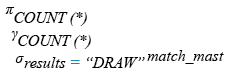Relational Algebra Tree: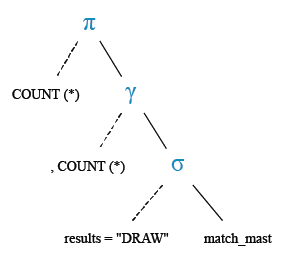## Practice Online

Sample Database: soccer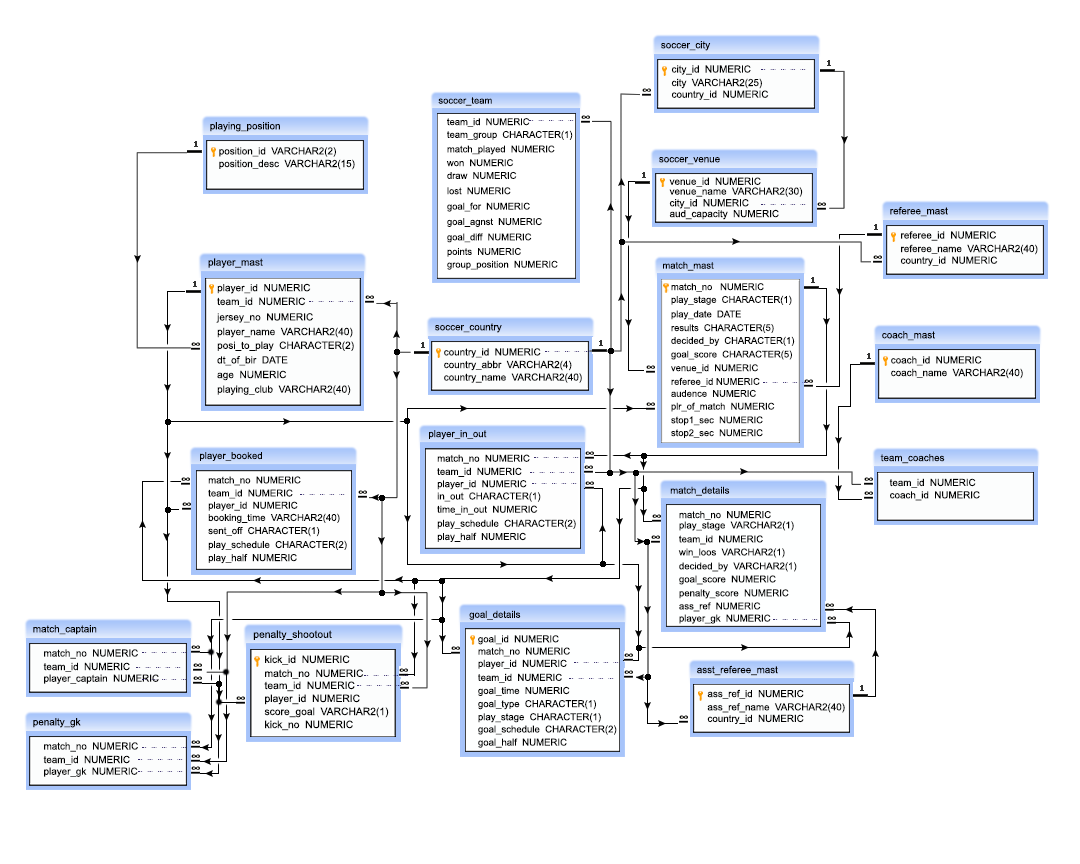## Query Visualization:

Duration: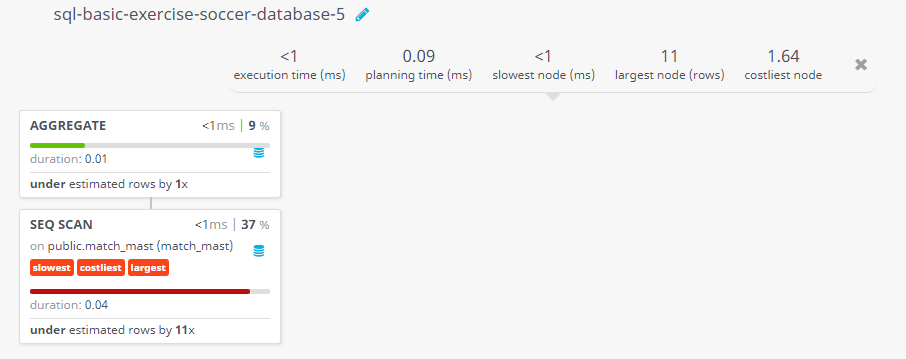Rows: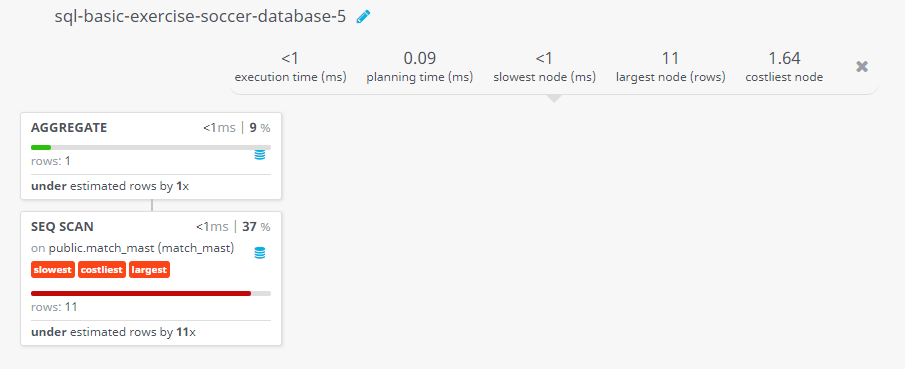Cost: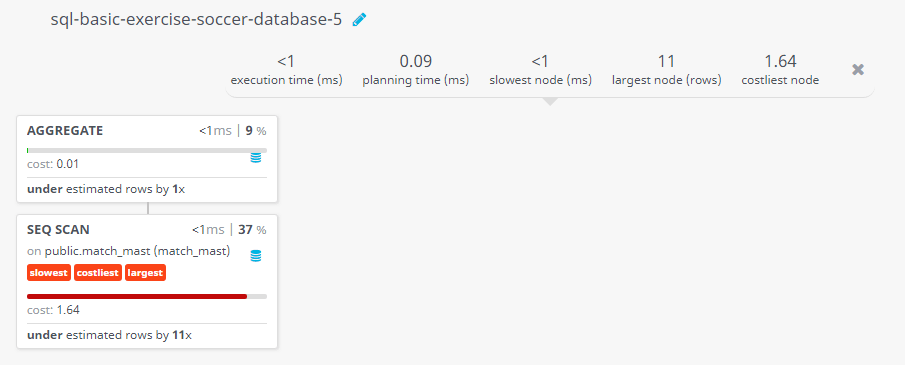Have another way to solve this solution? Contribute your code (and comments) through Disqus.

Previous SQL Exercise: Find the number of matches ended with a result.
Next SQL Exercise: Find the date when did Football EURO cup 2016 begin.

What is the difficulty level of this exercise?

Test your Programming skills with w3resource's quiz.

﻿